Solving a Linear - Quadratic System

A quadratic equation is defined as an equation in which one or more of the terms is squared but raised to no higher power.  The general form is ax2 + bx + c = 0, where a, b and c are constants.

 Linear - quadratic system: (where the quadratic is in one variable - only one variable is squared)

In a linear- quadratic system where only one variable in the quadratic is squared, the graphs will be a parabola and a straight line.  When graphing a parabola and a straight line on the same set of axes, three situations are possible.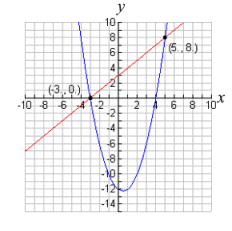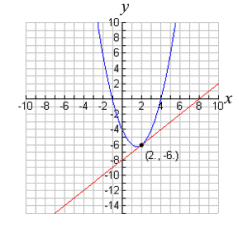The equations will intersect in two locations.  Two real solutions. The equations will intersect in one location.  One real solution. The equations will not intersect. No real solutions.

 Solve graphically: y = -x2 + 2x + 4  (quadratic - parabola) x + y = 4  (linear)
 1 Change the linear equation to "y=" form. y = -x + 4 2 Enter the equations as "y1=" and "y2=". (Be sure to use the negative key, not the subtraction key, for entering negative values.)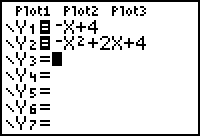3 Hit GRAPH to see if and where the graphs intersect. (Using ZOOM #6: ZStandard creates a 10 x 10 viewing window. You may need to adjust the WINDOW to see a clear picture of the intersection locations for the two graphs.)4 Under CALC (2nd Trace) choose #5 intersect to find the points where the graphs intersect.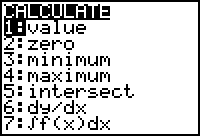5 When prompted for the "First curve?", move the spider on, or near, a point of intersection.  Hit Enter.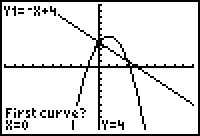6 When prompted for the "Second curve?", just hit Enter.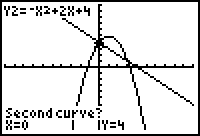7 Ignore the prompt for "Guess?", and hit Enter.8 Read the answers as to the coordinates of the point of intersection.  These coordinates appear at the bottom of the screen. Point of intersection (left side):  (0,4)9 If your graphs have a second point of intersection, repeat this process to find the second point.  Choose the #5 intersect choice and repeat the steps for finding the intersection. Point of intersection (right side):  (3,1)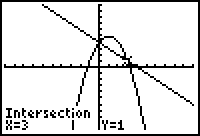Linear - quadratic system: (where the quadratic is in two variables - both variables are squared)

 Solve graphically: x2 + y2 = 25  (quadratic - circle) x - y = 5  (linear)
1. Change the linear equation to "y=" form.    y = x - 5
2. Change the quadratic equation to "y=" form.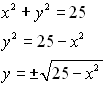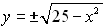can be entered as two separate entries:or a "list" notation may be used: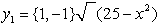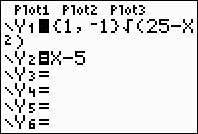Enter the equations.
The circle entry shown here uses the "list" notation.

3.
 If you hit GRAPH at this point using a standard window (ZOOM #6: ZStandard) your circle will not resemble a circle due to the 3 to 2 aspect ratio of this screen.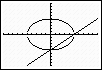Choose ZOOM #5: ZSquare to get a true looking circle.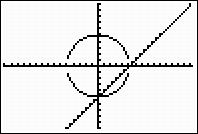Notice the missing pixels near the x-axis.

4. Under CALC (2nd Trace) choose #5 intersect to find the points where the graphs intersect.5. We will find the left point of intersection first.  When prompted for the "First curve?", use the down arrow key to move to the bottom portion of the circle.  Hit Enter.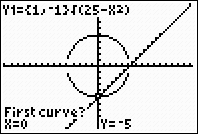6. When prompted for the "Second curve?", just hit Enter.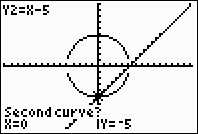7. Ignore the prompt for "Guess?", and hit Enter.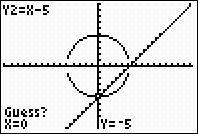8. Read the answers as to the coordinates of the point of intersection.  These coordinates appear at the bottom of the screen.

Point of intersection (left side):  (0,-5)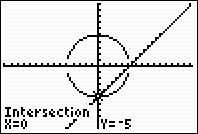9. We will find the right point of intersection next.  This point is occurring where the missing pixels are located.  We need to zoom in at this location to place more pixels on the screen.

After zooming, go to CALC (2nd Trace), choose #5 intersect, and find the points where the graphs intersect.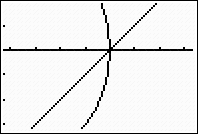10.30b
Notice the graph is set to Y1.    Use up and down arrows to get Y2.No need to guess.  Hit ENTER.
11. Point of intersection (right side):  (5,0)When working with a circle, always check the equation statement in the upper left corner to see where you are.  The up and down arrows will move you between the equations.Finding Your Way Around TABLE of  CONTENTS